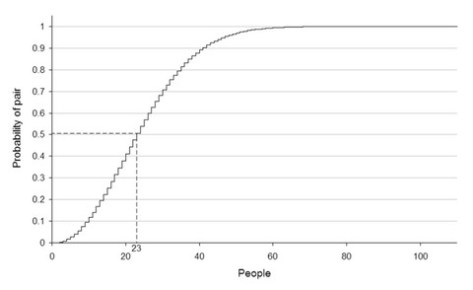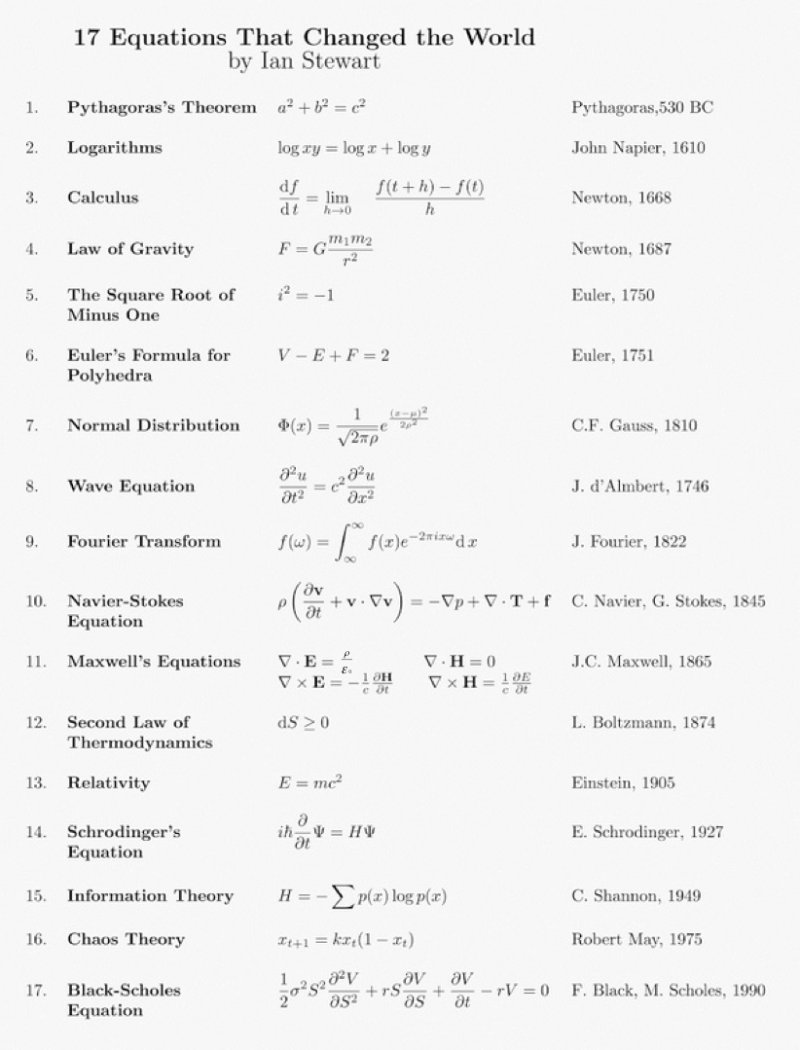### Problem Set 2 (2nd of 2) helpful hints)

The first set of problem set 2 hints appears at http://derivatives.garven.com/2023/01/25/problem-set-2-helpful-hints/.

Here’s the second problem set 2 hint, based on the following questions:

Finance 4366 Student Question: “I am in the process of completing Problem Set 2, but I am getting stumped, are there any class notes that can help me with 1E and question 2? I am having trouble finding the correct formulas. Thank you.”

Dr. Garven Answer (for question 2): Yesterday, I provided some hints about problem set #2 @ http://derivatives.garven.com/2023/01/25/problem-set-2-helpful-hints/.  Regarding question #2, here is what I wrote there: “The second problem involves using the standard normal probability distribution to calculate the probabilities of earning various levels of return by investing in risky securities and portfolios; see pp. 15-21 of the http://fin4366.garven.com/spring2023/lecture4.pdf lecture note for coverage of that topic.”

The inputs for this problem are all specified in parts A and B; in part A, expected return is 12% and standard deviation is 30%, whereas in part B,  expected return is 7.5% and standard deviation is 15%.  The probability of losing money in parts A and B requires calculating the z statistics for both cases.  In part A, the z stat is z = (0-mu)/sigma= (0-12)/30, and in part B it is z = (0-mu)/sigma= (0-7.5)/15.  Once you have the z stats, you can obtain probabilities of losing money in Parts A and B by using the z table from the course website (or better yet, your own z table).

Part C asks a different probability question – what the probability of earning more than 6% is, given the investment alternatives described in parts A and B.  To solve this, calculate 1-N(z) using the z table, where z = (6-mu)/sigma.

Dr. Garven Answer (for question 1E): Regarding 1E – since returns on C and D are uncorrelated, this means that they are statistically independent of each other.  Thus, the variance of an equally weighted portfolio consisting of C and D is simply the weighted average of these securities’ variances.  See the http://fin4366.garven.com/spring2023/lecture3.pdf lecture note, page 9, the final two bullet points on that page.  Also see the portfolio variance equation on page 13, which features two variance terms and one covariance term – Since C and D are statistically independent, the third term there (2w(1)w(2)sigma(12)) equals 0.

### The Birthday Paradox: an interesting probability problem involving “statistically independent” events

During this week’s statistics tutorials, we discussed (among other things) the concept of statistical independence, and focused attention on some important implications of statistical independence for probability distributions such as the binomial and normal distributions.

Here, I’d like to call everyone’s attention to an interesting (non-finance) probability problem related to statistical independence. Specifically, consider the so-called “Birthday Paradox”. The Birthday Paradox pertains to the probability that in a set of randomly chosen people, some pair of them will have the same birthday. Counter-intuitively, in a group of 23 randomly chosen people, there is slightly more than a 50% probability that some pair of them will both have been born on the same day.

To compute the probability that two people in a group of n people have the same birthday, we disregard variations in the distribution, such as leap years, twins, seasonal or weekday variations, and assume that the 365 possible birthdays are equally likely. Thus, we assume that birthdates are statistically independent events. Consequently, the probability of two randomly chosen people not sharing the same birthday is 364/365. According to the combinatorial equation, the number of unique pairs in a group of n people is n!/2!(n-2)! = n(n-1)/2. Assuming a uniform distribution (i.e., that all dates are equally probable), this means that the probability that no pair in a group of n people shares the same birthday is equal to p(n) = (364/365)^[n(n-1)/2]. The event of at least two of the n persons having the same birthday is complementary to all n birthdays being different. Therefore, its probability is p’(n) = 1 – (364/365)^[n(n-1)/2].

Given these assumptions, suppose that we are interested in determining how many randomly chosen people are needed in order for there to be a 50% probability that at least two persons share the same birthday. In other words, we are interested in finding the value of n which causes p(n) to equal 0.50. Therefore, 0.50 = (364/365)^[n(n-1)/2]; taking natural logs of both sides and rearranging, we obtain (ln 0.50)/(ln 364/365) = n(n-1)/2. Solving for n, we obtain 505.304 = n(n -1); therefore, n is approximately equal to 23.

The following graph illustrates how the probability that a pair of people share the same birthday varies as the number of people in the sample increases:It is worthwhile noting that real-life birthday distributions are not uniform since not all dates are equally likely. For example, in the Northern Hemisphere, many children are born in the summer, especially during the months of August and September. In the United States, many children are conceived around the holidays of Christmas and New Year’s Day. Also, because hospitals rarely schedule C-sections and induced labor on the weekend, more Americans are born on Mondays and Tuesdays than on weekends; where many people share a birth year (e.g., a class in a school), this creates a tendency toward particular dates. Both of these factors tend to increase the chance of identical birthdates since a denser subset has more possible pairs (in the extreme case when everyone was born on three days of the week, there would obviously be many identical birthdays!).

 Note that since 15 students are enrolled in Finance 4366 this semester, the probability that at least two Finance 4366 students share the same birthday is p’(15) = 1 – (364/365)^[15(14)/2] = 25%; this probability can also be inferred from the above figure.

### Z Table Extra Credit Assignment (due at the start of class on Tuesday, January 31)

Here’s an extra credit opportunity for Finance 4366. Working on your own (i.e., this is not a group project; I will only give credit for spreadsheets that are uniquely your own), build your own “z” table in Excel (patterned after the table at http://fin4366.garven.com/stdnormal.pdf); the top row should have values ranging from 0.00 to 0.09, and the first column should have z values ranging from -3.0 to +3.0, in increments of 0.1).

Conveniently, Excel has the standard normal distribution function built right in; e.g., if you type “=normsdist(z)”, Excel returns the probability associated with whatever z value you provide. If you type “=normsdist(0)”, .5 is returned, since half of the area under the curve lies to the left of the expected value E(z) = 0. Similarly, if you type “=normsdist(1)”, then .8413 is returned because 84.13% of the area under the curve lies to the left of z = 1. Perhaps you recall from your QBA course that 68.26% of the area under the curve lies between z = -1; this “confidence interval” of +/- 1 standard deviation away from the mean (E(z)=0) is calculated in Excel with the following code: “=normsdist(1)-normsdist(-1)”, and so forth.

You can turn your spreadsheet for this extra credit assignment in at the link labeled “Z Table Extra Credit Assignment” under the Assignment tab on Canvas.

### Problem Set 2 helpful hints

Problem Set 2 is available from the course website at http://fin4366.garven.com/spring2023/ps2.pdf; its due date is Tuesday, January 31.

Problem Set 2 consists of two problems. The first problem requires calculating expected value, standard deviation, and correlation, and using this information as inputs into determining expected returns and standard deviations for 2-asset portfolios. The second problem involves using the standard normal probability distribution to calculate the probabilities of earning various levels of return by investing in risky securities and portfolios; see pp. 15-21 of the http://fin4366.garven.com/spring2023/lecture4.pdf lecture note for coverage of that topic.

### Formula Sheet for today’s class problem in Finance 4366

As promised, I have posted the formula sheet for today’s statistics class problem in Finance 4366 at http://fin4366.garven.com/spring2023/statformulasheet.pdf. I highly recommend that everyone work through the class problem with the help of this formula sheet prior to Thursday’s Finance 4366 class meeting.

### On the ancient origin of the word “algorithm”

The January 24th assigned reading entitled “The New Religion of Risk Management” (by Peter Bernstein, March-April 1996 issue of Harvard Business Review) provides a succinct synopsis of the same author’s 1996 book entitled “Against the Gods: The Remarkable Story of Risk“. Here’s a fascinating quote from page 33 of “Against the Gods” which explains the ancient origin of the word “algorithm”:

“The earliest known work in Arabic arithmetic was written by al­Khowarizmi, a mathematician who lived around 825, some four hun­dred years before Fibonacci. Although few beneficiaries of his work are likely to have heard of him, most of us know of him indirectly. Try saying “al­Khowarizmi” fast. That’s where we get the word “algo­rithm,” which means rules for computing.”

Note: The book cover shown above is a copy of a 1633 oil-on-canvas painting by the Dutch Golden Age painter Rembrandt van Rijn.

### The 17 equations that changed the course of history (spoiler alert: we use 4 of these equations in Finance 4366!)

I especially like the fact that Ian Stewart includes the famous Black-Scholes equation (equation #17) on his list of the 17 equations that changed the course of history; Equations (2), (3), (7), and (17) play particularly important roles in Finance 4366!From Ian Stewart’s book, these 17 math equations changed the course of human history.

### Origin of the “Product Rule”, and Visualizing Taylor polynomial approximations

This blog entry provides a helpful follow-up for a couple of calculus-related topics that we covered during today’s Mathematics Tutorial.

1. See page 12 of the above-referenced lecture note.  There, the equation for a parabola ($y = {x^2}$) appears, and the claim that$\frac{{dy}}{{dx}} = 2x$ is corroborated by solving the following expression:In the 11-minute Khan Academy video at https://youtu.be/HEH_oKNLgUU, Sal Kahn takes on the solution of this problem in a very succinct and easy-to-comprehend fashion.
2. In his video lesson entitled “Visualizing Taylor polynomial approximations”, Sal Kahn replicates the tail end of today’s Finance 4366 class meeting in which we approximated y = ex with a Taylor polynomial centered at x=0 (as also shown in pp. 18-23 of the Mathematics Tutorial lecture note).  Sal approximates y = ex with a Taylor polynomial centered at x=3 instead of x=0, but the same insight obtains in both cases, which is that the accuracy of Taylor polynomial approximations increases as the order of the polynomial increases.